# Split Pandas Dataframe by Rows

In this article, we will explore the process of Split Pandas Dataframe by Rows. The Pandas DataFrame serves as the focal point, and throughout this discussion, we will experiment with various methods to Split Pandas Dataframe by Rows.

## Split Pandas DataFrame by Rows

In this article, we will elucidate several commonly employed methods for Split Pandas Dataframe by Rows. These methods are widely utilized for the purpose of dividing a Pandas DataFrame, and we will discuss the which are following:

• Using Row Index
• In Groups From Unique Column Values
• Using Predetermined-Sized Chunks
• By Shuffling Rows

Consider a dataset: To illustrate, let’s consider a dataset featuring information about diamonds.

## Python3

 `# importing libraries` `import` `seaborn as sns` `import` `pandas as pd` `import` `numpy as np`   `# data needs not to be downloaded separately` `df  ``=` `sns.load_dataset(``'diamonds'``)` `df.head()`

Output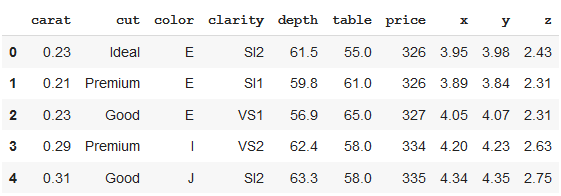### Splitting Pandas Dataframe by row index

In the below code, the dataframe is divided into two parts, first 1000 rows, and remaining rows. We can see the shape of the newly formed dataframes as the output of the given code.

## Python3

 `# splitting dataframe by row index` `df_1 ``=` `df.iloc[:``1000``,:]` `df_2 ``=` `df.iloc[``1000``:,:]` `print``(``"Shape of new dataframes - {} , {}"``.``format``(df_1.shape, df_2.shape))`

Output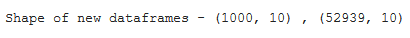### Splitting Pandas Dataframe by groups formed from unique column values

Here, we will first grouped the data by column value “color”. The newly formed dataframe consists of grouped data with color = “E”.

## Python3

 `# splitting dataframe by groups` `# grouping by particular dataframe column` `grouped ``=` `df.groupby(df.color)` `df_new ``=` `grouped.get_group(``"E"``)` `df_new`

Output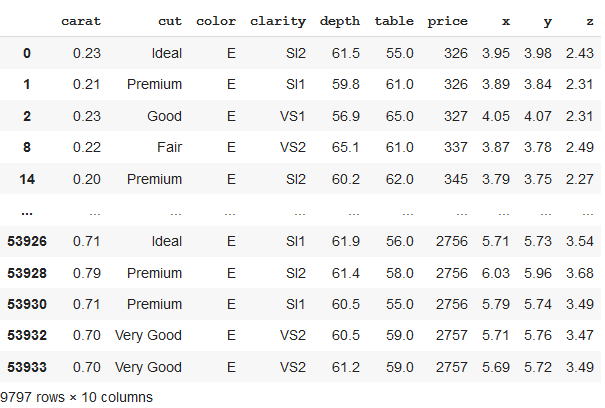### Splitting Pandas Dataframe in predetermined sized chunks

In the above code, we can see that we have formed a new dataset of a size of 0.6 i.e. 60% of total rows (or length of the dataset), which now consists of 32364 rows. These rows are selected randomly.

## Python3

 `# splitting dataframe in a particular size` `df_split ``=` `df.sample(frac``=``0.6``,random_state``=``200``)` `df_split.reset_index()`

Output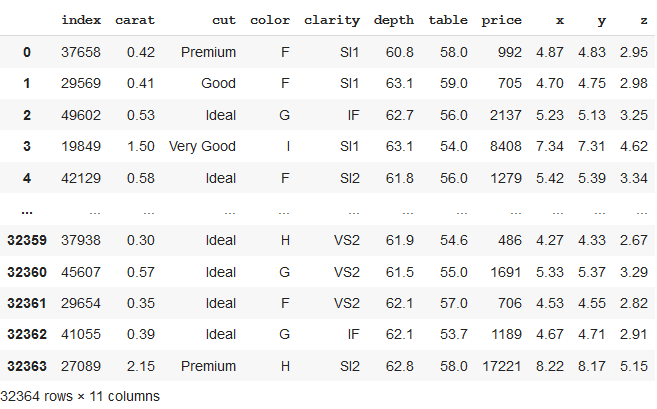### Split Pandas Dataframe by Shuffling Rows in Pandas DataFrame

In the below code , code randomly selects 50% of rows from DataFrame `df` into a new DataFrame `df1`. It then prints `df1` and its index-reset version using `reset_index()`.

## Python3

 `# Split DataFrame using sample()` `df1 ``=` `df.sample(frac ``=` `0.5``, random_state ``=` `200``)` `print``(df1)` `print``(df1.reset_index())`

Output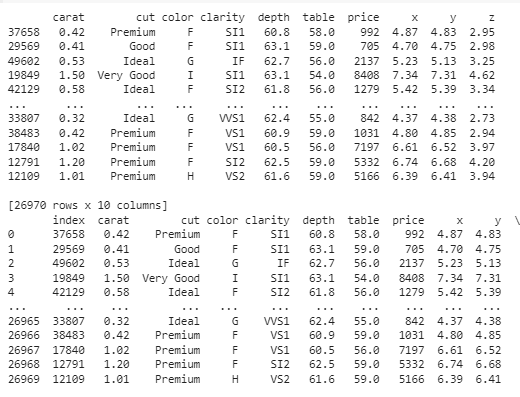### Conclusion

In summary, splitting Pandas DataFrames by rows offers a flexible way to organize and analyze data, allowing exploration of subsets through methods like random sampling or predetermined chunk sizes. This capability is valuable for tasks such as training machine learning models, exploratory data analysis, and efficient data processing, enhancing data manipulation in the field of data science.

Whether you're preparing for your first job interview or aiming to upskill in this ever-evolving tech landscape, GeeksforGeeks Courses are your key to success. We provide top-quality content at affordable prices, all geared towards accelerating your growth in a time-bound manner. Join the millions we've already empowered, and we're here to do the same for you. Don't miss out - check it out now!

Previous
Next